##### Trigonometry Workbook For DummiesYou can use L’Hôpital’s rule to find limits of sequences. L'Hôpital's rule is a great shortcut for when you do limit problems. Here it is: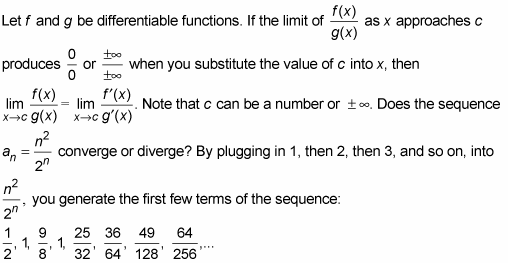Convergence and Divergence: You say that a sequence converges if its limit exists, that is, if the limit of its terms equals a finite number. Otherwise, the sequence is said to diverge.

What do you think? After going up for a couple terms, the sequence goes down and it appears that it’ll keep going down — looks like it will converge to zero. L’Hôpital’s rule proves it. You use the rule to determine the limit of the function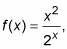which goes hand in hand with the sequence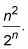Keep in mind that to use L’Hôpital’s rule, you take the derivative of the numerator and the derivative of the denominator, and then you replace the numerator and denominator by their respective derivatives.

For this problem, you have to use L’Hôpital’s rule twice: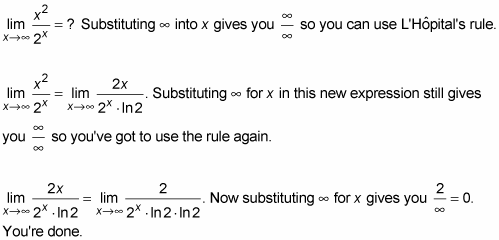Because the limit of the function is 0, so is the limit of the sequence, and thus the sequence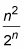converges to zero.